Ex 2.3

Chapter 2 Class 10 Polynomials
Serial order wise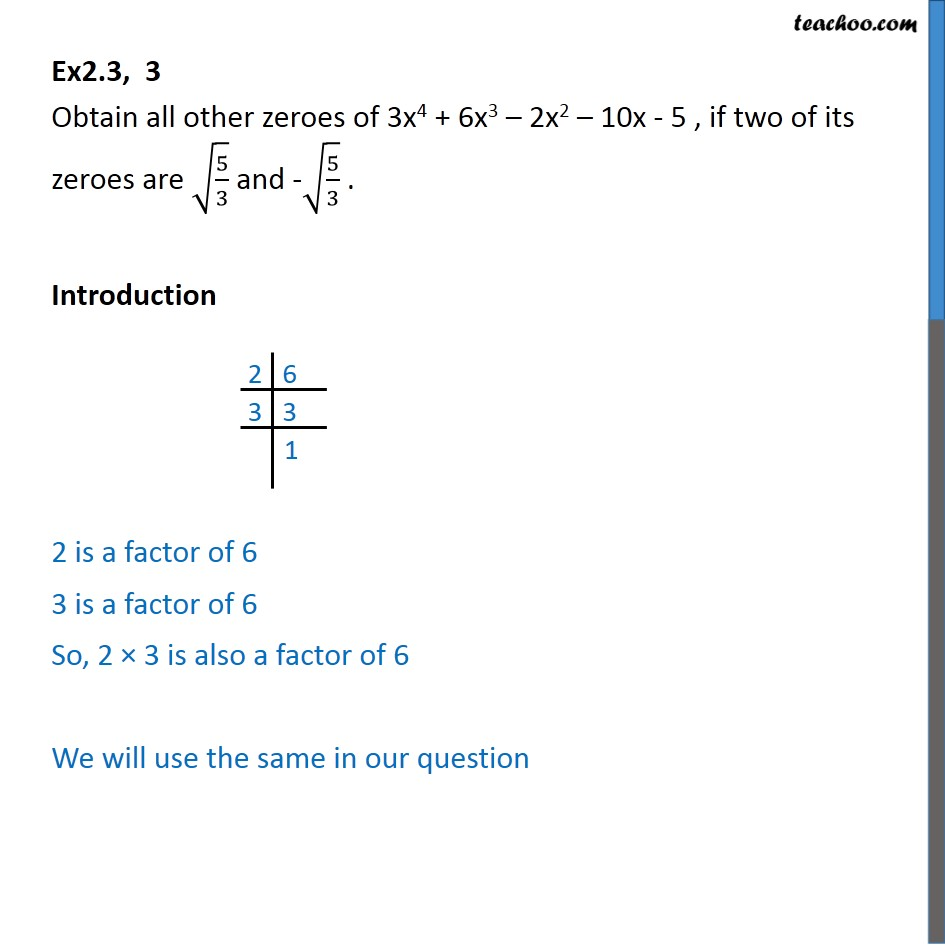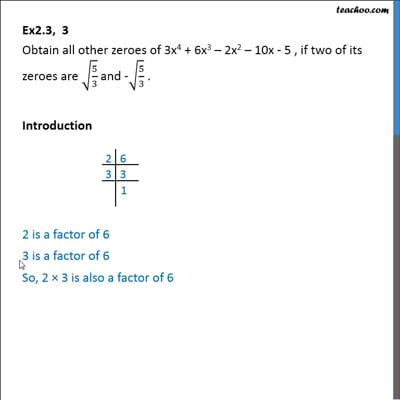This video is only available for Teachoo black users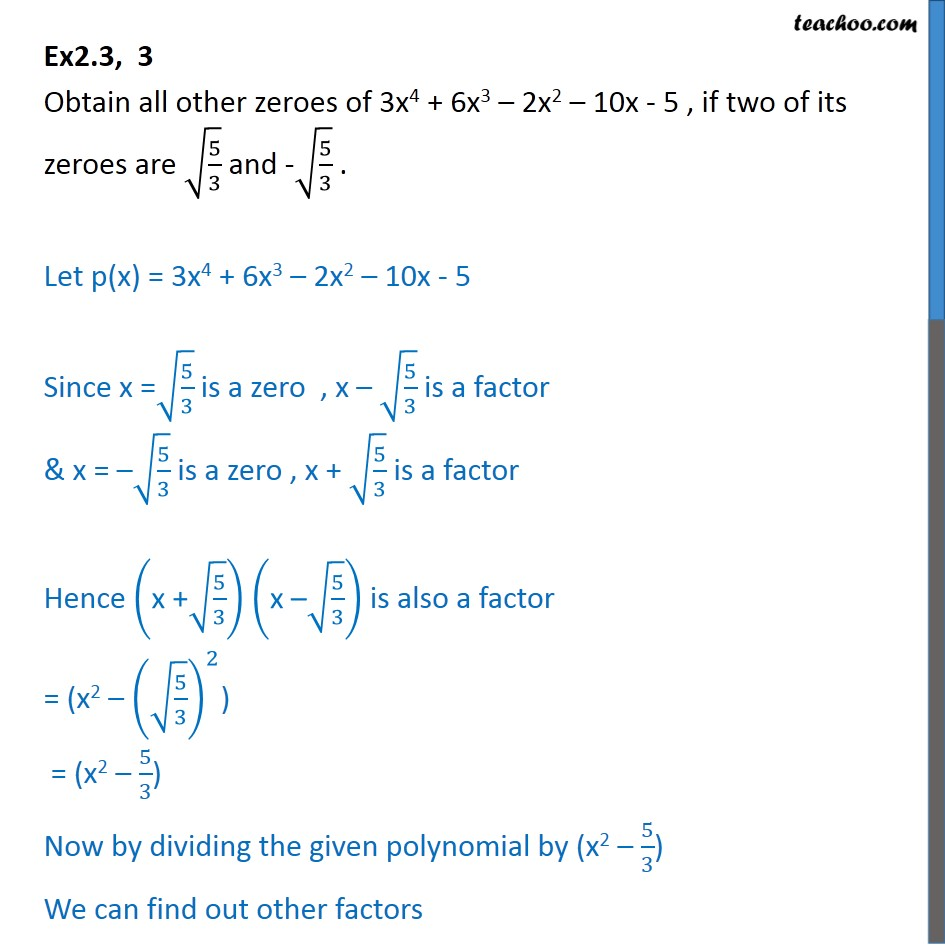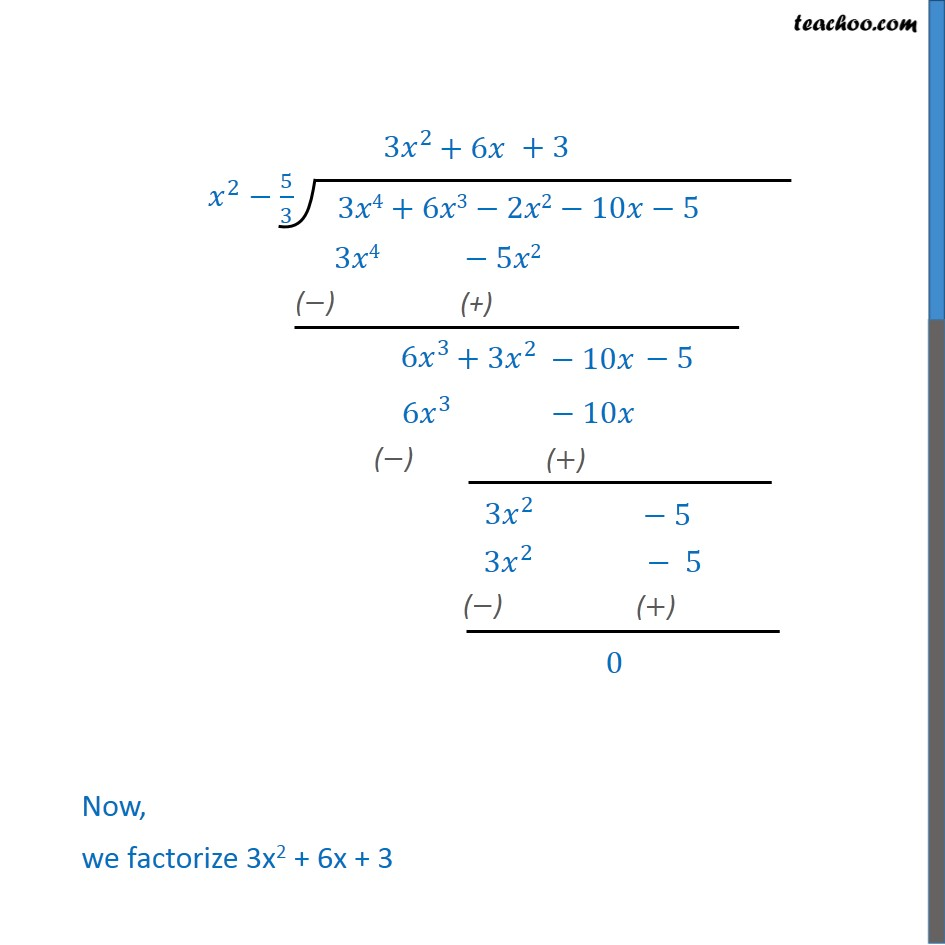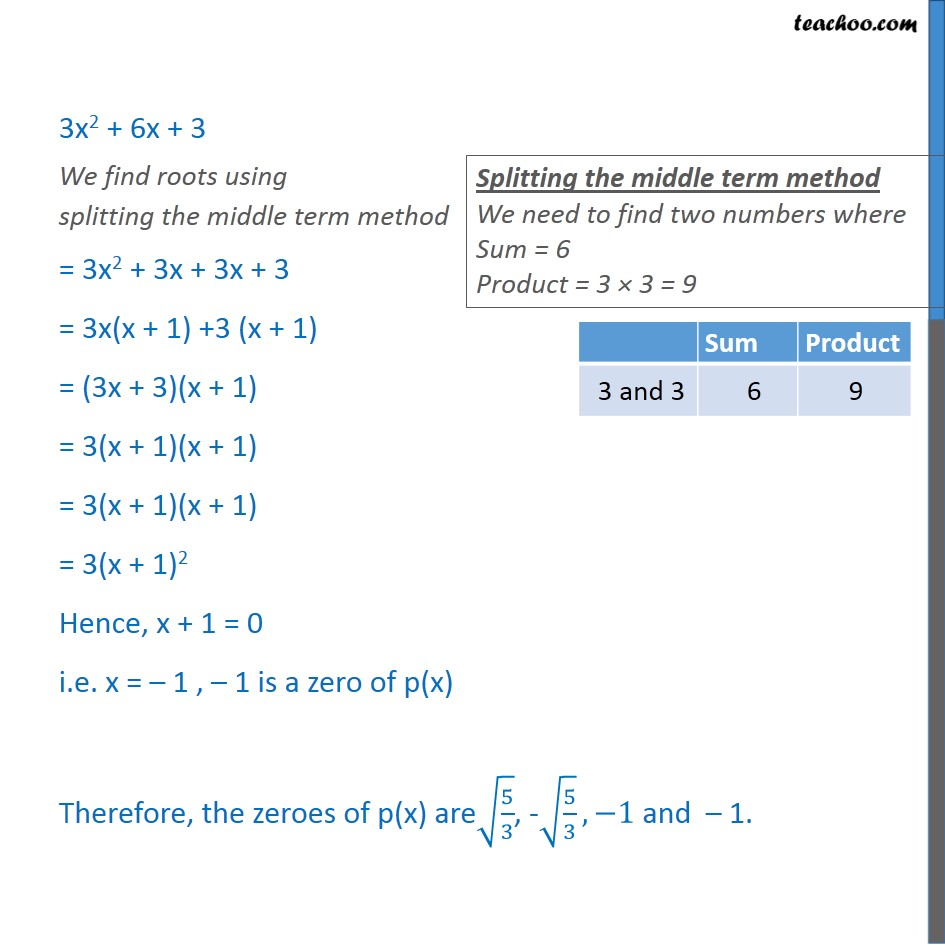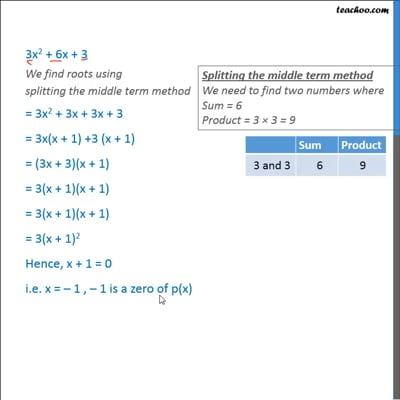This video is only available for Teachoo black users

Introducing your new favourite teacher - Teachoo Black, at only ₹83 per month

### Transcript

Ex2.3, 3 Obtain all other zeroes of 3x4 + 6x3 – 2x2 – 10x - 5 , if two of its zeroes are √(5/3) and -√(5/3) . Introduction 2 is a factor of 6 3 is a factor of 6 So, 2 × 3 is also a factor of 6 We will use the same in our question Ex2.3, 3 Obtain all other zeroes of 3x4 + 6x3 – 2x2 – 10x - 5 , if two of its zeroes are √(5/3) and -√(5/3) . Let p(x) = 3x4 + 6x3 – 2x2 – 10x - 5 Since x =√(5/3) is a zero , x – √(5/3) is a factor & x = –√(5/3) is a zero , x + √(5/3) is a factor Hence ("x +" √(5/3)) ("x –" √(5/3)) is also a factor = (x2 – (√(5/3))^2) = (x2 – 5/3) Now by dividing the given polynomial by (x2 – 5/3) We can find out other factors Now, we factorize 3x2 + 6x + 3 3x2 + 6x + 3 We find roots using splitting the middle term method = 3x2 + 3x + 3x + 3 = 3x(x + 1) +3 (x + 1) = (3x + 3)(x + 1) = 3(x + 1)(x + 1) = 3(x + 1)(x + 1) = 3(x + 1)2 Hence, x + 1 = 0 i.e. x = – 1 , – 1 is a zero of p(x) Therefore, the zeroes of p(x) are√(5/3), -√(5/3), −1 and – 1.